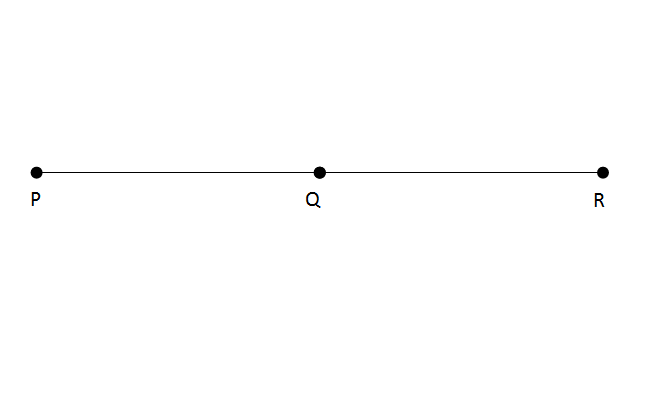# CAT Quantitative Aptitude Questions | Geometry Questions for CAT - Triangles

###### CAT Questions | CAT Geometry Questions | Lines

The question is from the topic lines. In this problem, it tests our understanding on basic properties of a line. CAT Geometry questions are heavily tested in CAT exam. Make sure you master Geometry problems.

Question 40: In the below figure, If $$frac{PR}{PQ}\\$ = $\frac{PQ}{QR}\\$, then1. $\frac{PQ}{QR}\\$ > 2 2. $\frac{PQ}{QR}\\$ = 2 3. $\frac{PQ}{QR}\\$ < 2 4. can’t be determined ## 🎉Get Upto ₹6,000 off on our CAT 2024 and IPM 2024/25 courses! Valid till 1st December 2023.★ Register now ## Best CAT Coaching in Chennai #### CAT Coaching in Chennai - CAT 2022Limited Seats Available - Register Now! ### Explanatory Answer ##### Method of solving this CAT Question from Triangles: It is a very basic question. Let's start with the given details.Given, $\frac{PR}{PQ}\\$ = $\frac{PQ}{RQ}\\$ Here, PR = PQ + RQ => 1 + $\frac{RQ}{PQ}\\$ = $\frac{PQ}{RQ}\\$ Let $\frac{PQ}{RQ}\\$ = x => 1+ $\frac{1}{x}\\$ = x => x+ 1 = x2 => x2 - x - 1 = 0 => x = $\frac{$+1±√(1+4$)}{2}$ = $\frac{1±√5}{2}\\$ , Since PQ – RQ > 0 => x = $\frac{1+√5}{2}\\$ which is between 1 and 2 The question is " If $\frac{PR}{PQ}\\$ = $\frac{PQ}{RQ}\\$, then " ##### Hence, the answer is $\frac{PQ}{QR}\\$ < 2 Choice C is the correct answer. ###### 🎉Get Upto ₹6,000 off on our CAT 2024 and IPM 2024/25 courses! Valid till 1st December 2023. ###### Already have an Account? ###### CAT Coaching in ChennaiCAT 2023 Classroom Batches Starting Now! @Gopalapuram ###### Best CAT Coaching in Chennai Introductory offer of 5000/- Attend a Demo Class ###### Best Indore IPM & Rohtak IPM CoachingSignup and sample 9 full classes for free. Register now! ## CAT Preparation Online | CAT Geometry questions Videos On YouTube #### Other useful sources for Geometry Question | Geometry Triangles Circles Quadrilaterals Sample Questions ##### Where is 2IIM located? 2IIM Online CAT Coaching A Fermat Education Initiative, 58/16, Indira Gandhi Street, Kaveri Rangan Nagar, Saligramam, Chennai 600 093 ##### How to reach 2IIM? Phone:$91) 44 4505 8484
Mobile: (91) 99626 48484
WhatsApp: WhatsApp Now
Email: prep@2iim.com